# Based on the figure shown in the exhibit, which letter represents the placement of the roughness...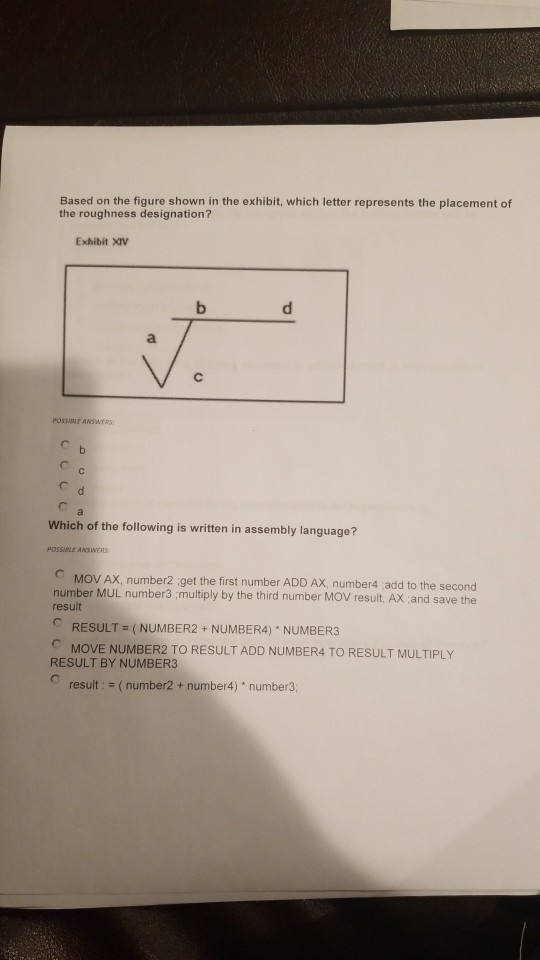Based on the figure shown in the exhibit, which letter represents the placement of the roughness designation? Exhibit XIv POSSIBLE ANSWYRS Which of the following is written in assembly language? POSSIBLE ANSWERS MOV AX, number2 get the first number ADD AX, number4 add to the second number MUL number3 multiply by the third number MOV result, AX and save the result CRESULT (NUMBER2 + NUMBER4) NUMBER3 MOVE NUMBER2 TO RESULT ADD NUMBER4 TO RESULT MULTIPLY RESULT BY NUMBER3 result: = ( number2 + number4)" number3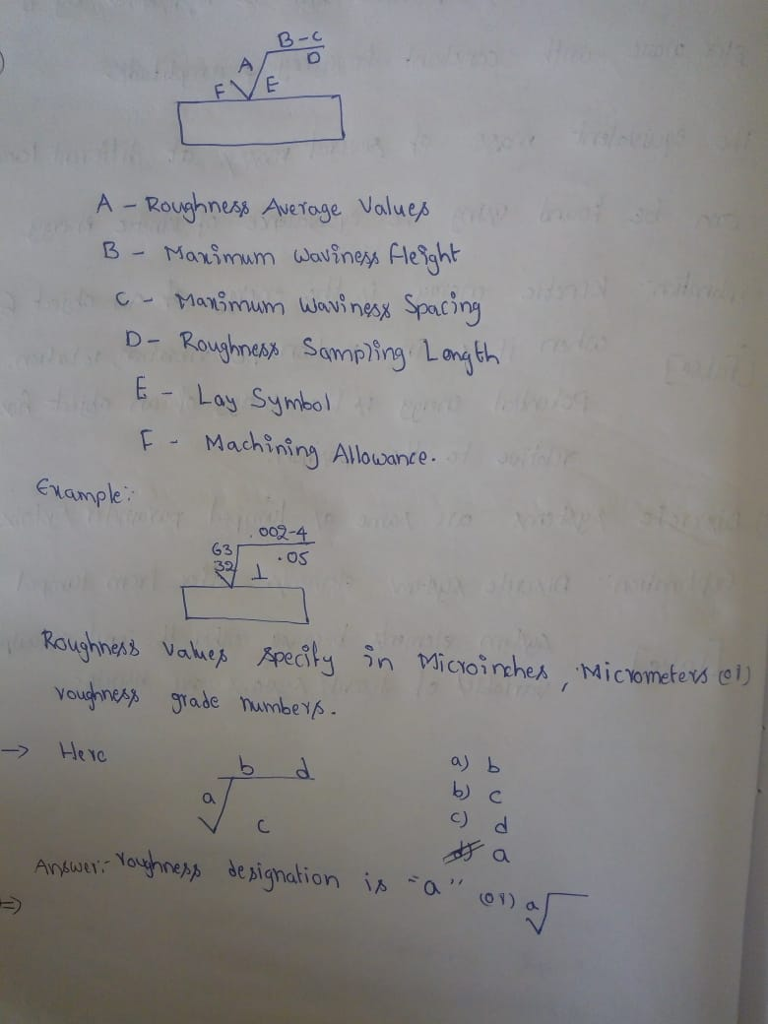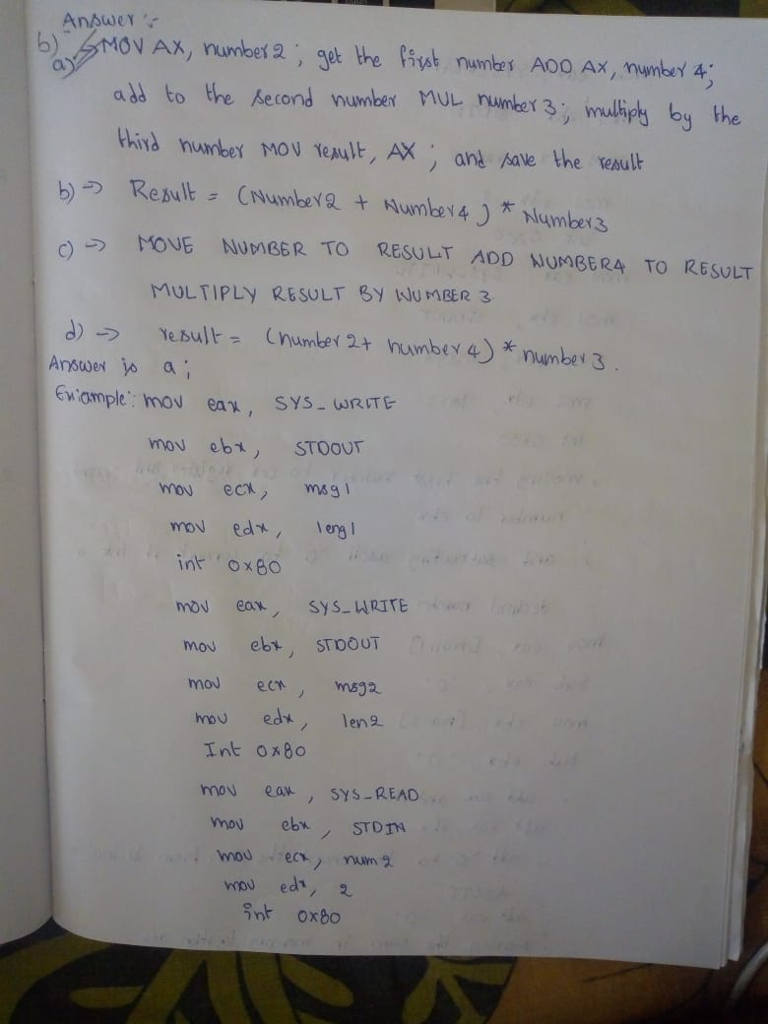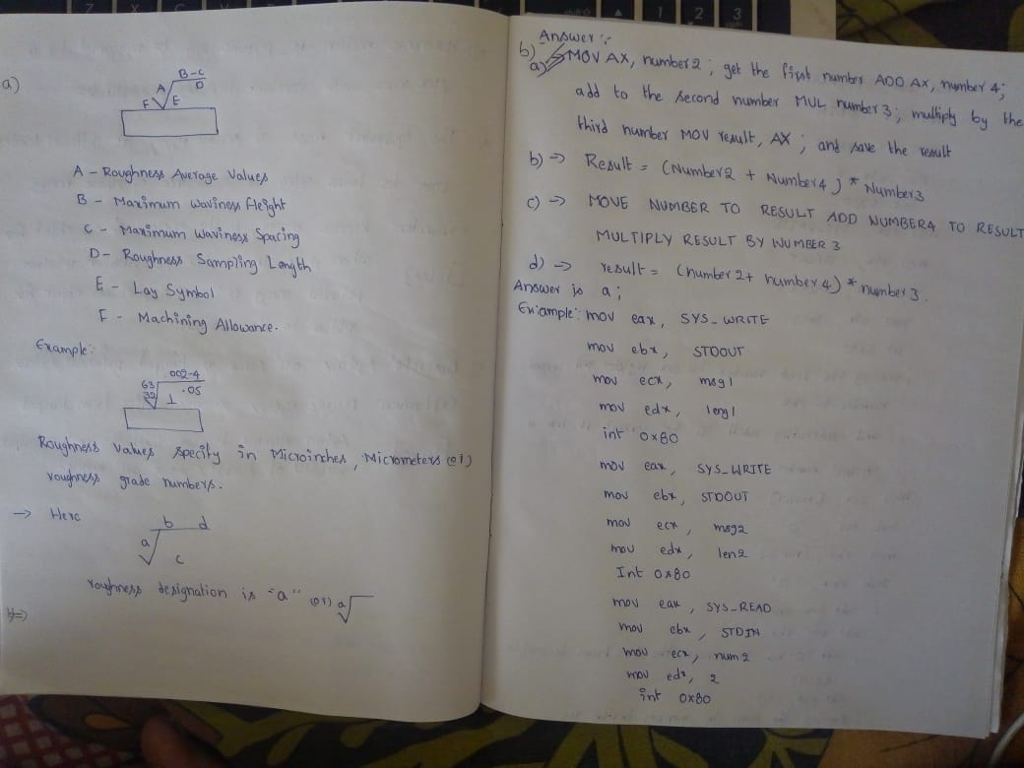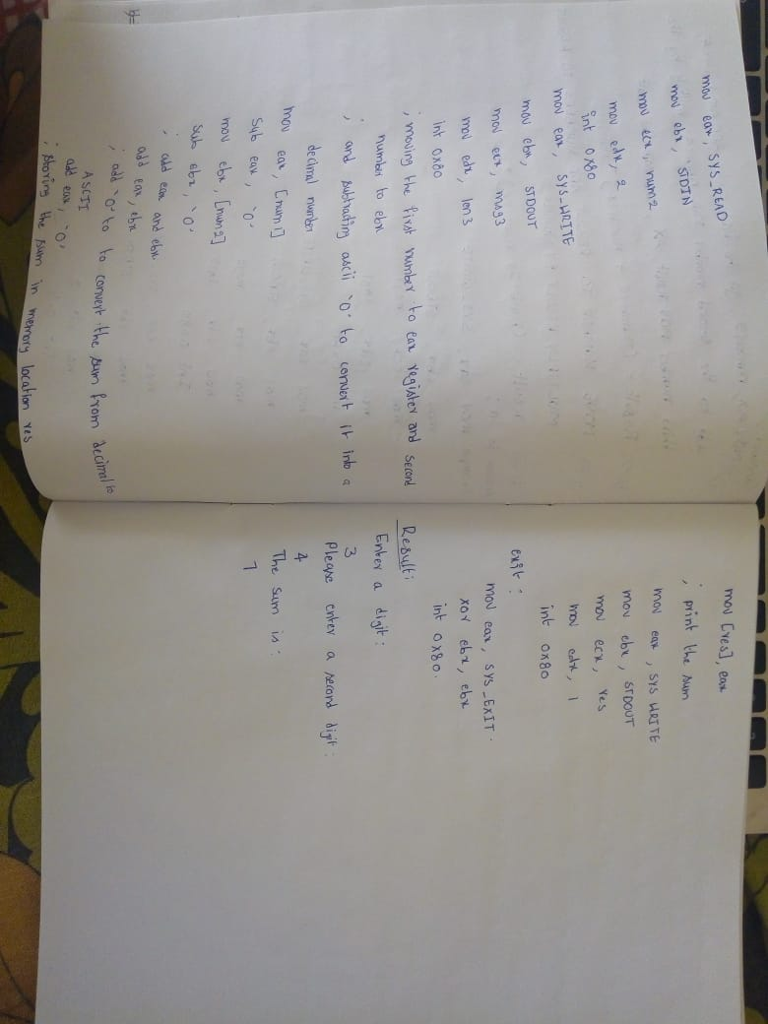##### Add Answer to: Based on the figure shown in the exhibit, which letter represents the placement of the roughness...
Similar Homework Help Questions
• ### We were unable to transcribe this imageBased on the figure shown in the exhibit, which letter...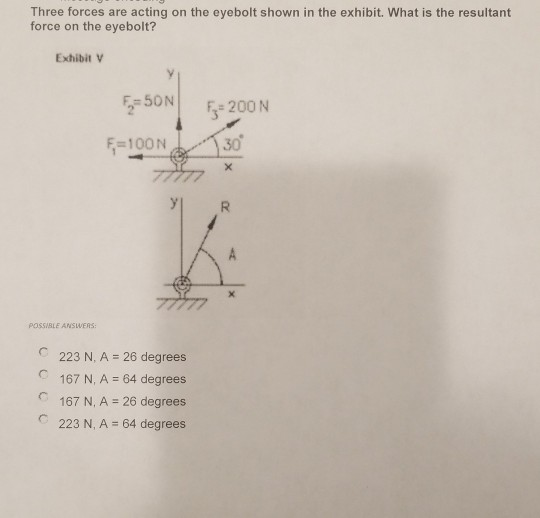We were unable to transcribe this imageBased on the figure shown in the exhibit, which letter represents the placement of the roughness designation? Exhibit XIV POSSIBLE ANSWERS: What feature control symbol should be specified in the figure shown in the exhibit? EXHIBIT XIII t 10.50 TOLERANCE SPECIFICATION 0.50 TOLERANCE ZONIE INTERPRETATION POSSIBLE ANSWERS Cylindricity Concentricity CStraightness Profile

• ### Assignment 4 File “quad_sol.s” contains a quadratic polynomial solver, which calculates the integer solution of a quadratic polynomial equation. 1. Rewrite the program using instructions reordering to...

Assignment 4 File “quad_sol.s” contains a quadratic polynomial solver, which calculates the integer solution of a quadratic polynomial equation. 1. Rewrite the program using instructions reordering to reduce the number of cycles needed to execute the program. Indicate the number of cycle reduction. 2. Describe how forwarding would affect the execution of the program. CODE # quad_sol.s # This assembly program calculates the integer solutions of a quadratic polynomial. # Inputs : The coefficients a,b,c of the equation a*x^2 +...

• ### I need help to develope my assignment please. here are rhe instructions: Minimum word count for each sub-heading ans...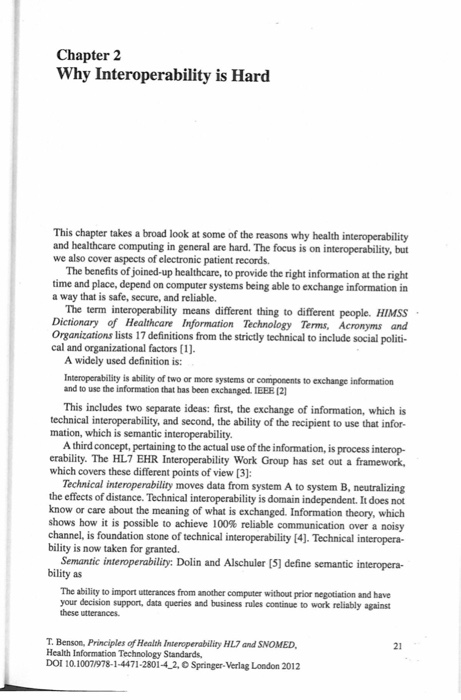I need help to develope my assignment please. here are rhe instructions: Minimum word count for each sub-heading answer is 50 words (for example #1 has three parts so your complete answer including a, b and c should be at least 150 words) be sure to cover all three questions completely in the answer. The chapter begins discussing technical, process and semantic interoperability and ends with change management. The sub-headings in the chapter include: 1. Why Standards are Needed-a) why...

Free Homework App